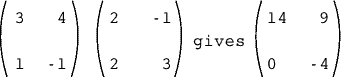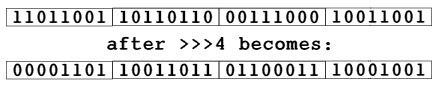BBC BASIC V A dabhand guide

### Chapter 2 : Operators

Our investigation of BASIC V starts with a comparatively low-key subject, but one which forms the core of so many instructions. All programming languages use a variety of operators. Obvious examples are the arithmetic operators such as multiply (*) and divide (/). There are also logical operators such as AND and OR, and there are relational operators such as 'greater than' (>) and 'not equal to' (<>).

Some symbols can have more than one meaning, determined by the context in which they are used. For example, the equals symbol (=) is used both as a relational operator (eg, IF x=y THEN ...) and as an assignment operator (eg, disc=b^2-4*a*c). Similarly the plus sign (+) is used both for the addition of numbers and the concatenation (joining together) or strings (A=B+C and A\$=B\$+C\$).

#### New Assignment Operators

BASIC V introduces a number of additional operators. There are two new assignment operators for incrementing or decrementing the values of variables. Examples are:

```     X+=1:Y+=1  : Increment X and Y by one.
X-=FNmouse : Decrement X by the value returned by FNmouse.```

The variable on the left of the 'plus/equals' (or 'minus/equals') sign is incremented (or decremented) by the value of the expression to the right of this sign. The examples above are equivalent to:

```     X=X+1:Y=Y+1
X=X-FNmouse```

There is one small but important difference between the two forms, the old and the new. On the Archimedes, a statement like:

`     X=X+1`

is sufficient to declare the variable X with an initial value of zero, which is then incremented. The new form:

`     X+=1`

does not initialise X, and unless this has been explicitly undertaken beforehand, an error will result ("Mistake").

#### New Unary Operators

Unary operators are those that operate on a single value rather than two, as do most operators. Minus (-) is a unary operator because we can write statements like:

`      X1=-X`

where the minus sign is applied to the value of x, and the resulting value is assigned to the variable X1. The minus sign, confusingly, can also be used as a binary operator, (binary meaning that it has two operands), when used to indicate the operation of subtraction. Other examples of unary operators that already exist are the tilde (~) and ampersand (&) for conversion to and from hexadecimal formats, and the so-called indirection operators (?, ! and \$), the first two of which can be used both as unary or binary operators.

BASIC V adds two new unary operators to those already available. These are 'per cent' (%) to indicate a binary value (just as & indicates hexadecimal) and 'bar' (|) to specify 5-byte floating-point indirection. Thus %101101 is the same as 45 (decimal) and &2D (hex). You will also find that ~%101101 will convert the binary format directly into a hexadecimal format (&2D). The main purpose of this operator is to allow constants to be specified in binary where this is more appropriate. For example, if you want to convert a character (entered as either upper or lower case) into upper case only, the following conversion will suffice:

`     char%=GET AND %11011111`

Since the AND is specifically required to operate in a bit-wise manner, specifying the second operand in binary makes much more sense. Unfortunately, there is no binary equivalent of the tilde (~) for conversion of numbers into a binary format for display and printing.

The other new unary operator extends the indirection operators to include floating point explicitly. Thus, given a suitable memory location (say P%), the following assignments may be made:

```     ?P%=123    byte - maximum value 255
!P%=32666   integer - standard four-byte format
!P%=3.1415927  floating point - standard five-byte
\$P%="Hello"  string - terminated by a zero```

Unlike the byte and integer indirection operators, the new floating-point indirection operator may only be used as a unary operator. Thus:

`     P%!5=3.1415927`

is illegal and will generate the error message "Mistake". However, there is no constraint on using the new operator in the form:

`     !(P%+5)=3.1415927`

This form, therefore, provides an even better alternative to the '!' operator for copying sections of memory from one location to another, by moving five rather than four bytes at a time.

#### New Binary Operators

As already explained, binary operators are those which require two operands, and are nothing in particular to do with the binary number system. BASIC V now provides three shift operators as follows:

```     X >> b  Arithmetic shift right
X >>> b  Logical shift right
X << b  Logical shift left```

The first operand is the value to be shifted, and the second specifies the number of bits. The User Guide gives scant information on the precise action performed by these operators and yet the detail of how they work is crucial. In principle, shifting 1 bit to the right is equivalent to dividing the number by 2, while shifting 1 bit to the left is equivalent to multiplying by the same amount.

When applying a shift, BASIC converts any number into a 32-bit format. These operations can be applied to floating point numbers and variables provided that their values do not go beyond the accepted integer range (-2147483648 to 214783647 decimal, &FFFFFFFF to &7FFFFFFF hex). For this reason it is best to confine these operations to integer variables and their values only. In any case, it makes little sense to apply what are essentially bit-wise operations to floating point numbers held in mantissa/exponent format (often called 'scientific' notation).

The arithmetic shift right preserves the sign, while the number, positive or negative, becomes smaller and smaller. This is illustrated in figure 2.1. If the operation is repeated indefinitely, then a steady state is ultimately reached with the smallest positive (0) or negative (-1) number that can be represented. Because BASIC uses a twos complement notation, -1 (decimal) is represented by an all ones value in binary (&FFFFFFFF in hex). Further arithmetic shifts to the right merely serve to preserve the existing number.Figure 2.1

Contrast this with what happens when a logical shift right is applied. This time the 32 bits are treated as an unsigned value and all bits are shifted to the right, with zero being introduced from the left. Repeated applications of the logical shift right will therefore always result ultimately in a zero value, regardless of the starting value or what it represents.Figure 2.2

There is only one shift left and this does not preserve the sign (if any), while zeros are shifted in from the right. Since a '1' in the left-most bit position indicates a negative number in twos complement notation, you are likely to find that any number apparently oscillates between positive and negative if a shift left is applied repeatedly.

The shortest way to try this out for yourself is to use the following in immediate mode and look at the results:

`     X%=45:REPEAT:P.~X%,X%:X%=X%>>1:UNTIL FALSE`

This will produce a list of both hexadecimal and decimal values as the value initially assigned to X% is progressively shifted one bit at a time. Just substitute whatever integer value you want to try in place of the 45 (and you will need to substitute both negative and positive values to see properly what is happening), and replace the '>>' by either of the other two shift operators ('>>>' and '<<') as you wish. Use ESCAPE to terminate execution.

A more sophisticated version of this routine, which prompts for the initial number and shift operator, is listed at the end of this chapter. The output is displayed in hexadecimal and decimal formats in order to provide the maximum information. Try both positive and negative numbers with all three types of shift operator in turn.

As a simple example of the application of shift operators in BASIC V, consider the selection of colours in the 256-colour modes. If the variables red%, green% and blue% each specify the amount of these primary colours (in the range of 0 to 3), then any one of 64 colours may be selected by writing:

`     COLOUR blue%<<4 + green%<<2 + red%`

The 64 colours may be expanded to 256 by the addition of tint, in which case the statement above becomes modified to:

`     COLOUR blue%<<4 + green%<<2 + red% TINT tint%<<4`

#### Swapping Variables

Although not strictly an operator, we complete this chapter by looking at the new SWAP command. This allows the values of two variables (including elements or arrays), or two complete arrays, to be swapped. The typical statement found in sort routines:

`     IF data(I%)>data(I%+1) THEN PROCswap(I%)`

where PROCswap is defined as:

```     DEF PROCswap(i%)
LOCAL temp
temp=data(i%):data(i%)=data(i%+1):data(i%+1)=temp
ENDPROC
```

can now be written very simply as:

`     IF data(I%)>data(I%+1) THEN SWAP data(I%),data(I%+1)`

Other examples of the SWAP statement could include:

```     SWAP name1\$,name2\$
SWAP newx,oldx
SWAP matrix1(),matrix2()
```

Values being swapped must both be of the same type (integer, floating point, or string). In the case of complete arrays, the number of subscripts and the upper limits of the two arrays are also swapped. Thus, if two arrays are dimensioned as:

`     DIM fred(20), freda(30,30)`

then, after executing SWAP fred(),freda(), it would be as though the two arrays had been dimensioned as:

`     DIM fred(30,30), freda(20)`

Listing 2.1. Demonstration of Shift Operations.

```     10 REM >Chap2-1
100 MODE 7:VDU14
110 PRINTTAB(12,1)"SHIFT OPERATORS"
120 VDU28,0,24,39,3
130 ON ERROR PROCerror:IF err% THEN END
140 DIM shift\$(3):shift\$(1)=">>"
150 shift\$(2)=">>>":shift\$(3)="<<"
160 REPEAT:CLS
170 INPUT''"Enter any integer value: " X%
180 PRINT'TAB(5)"1. Arithmetic shift right"
190 PRINT'TAB(5)"2. Logical shift right"
200 PRINT'TAB(5)"3. Shift left
210 INPUT'"Enter operation required (1, 2, 3): " P%
220 PRINT'TAB(5)"Hex"TAB(23)"Decimal"'
230 REPEAT
240 PRINT ~X%,SPC7,X%
250 X%=EVAL(STR\$(X%)+shift\$(P%)+"1")
260 UNTIL X%=0 OR X%=-1
270 PRINT ~X%,SPC7,X%
280 PRINT "Press any key to continue";:G=GET
290 UNTIL FALSE
300 END
310 :
320 DEF PROCerror:err%=FALSE
330 IF ERR<>20 THEN
340 err%=TRUE
350 PRINT REPORT\$;" at line ";ERL
360 ENDIF
370 ENDPROC
```

© Alligata Media 2015